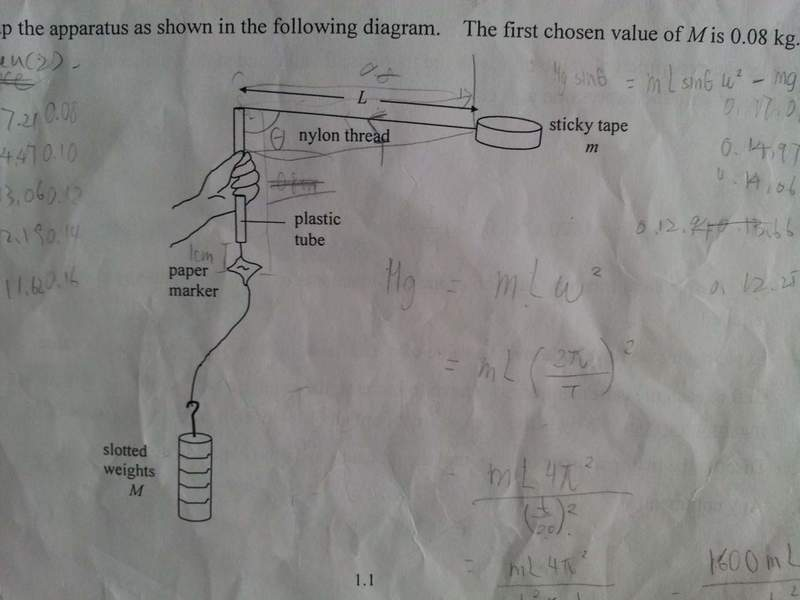# Centripetal acceleration graphMg=[1600∏2m(L+a)(1/t2)]- b

M is the mass of the slotted weight
g is the gravitional force
m is the mass of the sticky tape
L is the length of the nylon thread measured from the end of the plastic tube to the sticky tape
t is the time for 20 revolutions of the sticky tape
a and b are constants

Excuse me, I would like to ask:
1. What are the physical meaning of a and b ?
2. How can I plotted a suitable graph to determine the value of g based on these datas?

Welcome to forum!

It is very difficult to understand the problem.Can you post the whole problem statement?

Welcome to forum!

It is very difficult to understand the problem.Can you post the whole problem statement?

It's an experiment to verify centripetal acceleration= rω2
Producedures:
1. Set up the apparatus as shown in the photo.

2.Hold the plastic tube vertically and whirl the sticky tape above your head in a horizontial circle.Increase the angular speedof the sticky tape to a maximum value such that the paper marker still remains stationary at the position 1cm below the lower end of the plastic tube.Keep the tape roating at that particular speed throughout the motion.

3. Mesure the t for 20 revolutions of the t.

4. Repeat the prodcedures by using different mass of M.

•1 person
is that equation you derived or it is given?

It is given ><

You should make a similar equation like the one that is given and compare the both.From this you will get what are a and b.

You should make a similar equation like the one that is given and compare the both.From this you will get what are a and b.

My work:
Fc= mrω2
Mg= mL4∏2 / (t/20)2
= 1600mL∏2 / t2

And I don't know why there are a and b :(

haruspex
Homework Helper
Gold Member
2020 Award
View attachment 64241

Mg=[1600∏2m(L+a)(1/t2)]- b

1. What are the physical meaning of a and b ?
Looks like a is to account for the radius of the tape. L is not the distance to its centre. 'a' should be slightly more than the radius.
'b' may be allowing for some static friction in the tube.
2. How can I plotted a suitable graph to determine the value of g based on these datas?
In the OP you said it was to verify the rω2 formula for centripetal force.
That means you need to show that there are values for a and b which make the observations fit well.
If you plot M (as y) against a suitable function of t as x you should get a straight line. What function of t?

•1 person
i think that here we have considered the string to be ideal but it is not.So a and b should be making the system ideal.

•1 person
Looks like a is to account for the radius of the tape. L is not the distance to its centre. 'a' should be slightly more than the radius.
'b' may be allowing for some static friction in the tube.

In the OP you said it was to verify the rω2 formula for centripetal force.
That means you need to show that there are values for a and b which make the observations fit well.
If you plot M (as y) against a suitable function of t as x you should get a straight line. What function of t?

But sorry, I don't quite understand the meaning of " What function of t?" ><

i think that here we have considered the string to be ideal but it is not.So a and b should be making the system ideal.

There are some hint in the questions.
It asked that why we hv to increase the angular speed of the tape to a maximum value, and how does it releate to the value of b?

There are some hint in the questions.
It asked that why we hv to increase the angular speed of the tape to a maximum value, and how does it releate to the value of b?

i agree with solution of haruspex.the constant b is the static friction of the plastic tube which is opposing the centripetal acceleration.Hence it is adding to the Mg .

i agree with solution of haruspex.the constant b is the static friction of the plastic tube which is opposing the centripetal acceleration.Hence it is adding to the Mg .

I also agree with that ^^

haruspex
•